# OEImage¶

```class OEImage : public OEImageBase
```

OEImage class is implemented as a display list i.e. a container of drawing commands. For example, when the `OEImage.DrawLine` method is called, rather than immediately drawing a line specified by the parameters, a line drawing operation is created that copies all parameters for later execution.

When the `OEImage.Render` method is invoked, the drawing commands stored in the OEImage object are executed in the same order in which they were issued.

The following methods are publicly inherited from OEImageBase:

## Constructors¶

```OEImage(double width, double height, const OESystem::OEColor& bgColor = OESystem::OEWhite);
```

Default constructor that creates an OEImage with the specified width and height.

width, height

The dimensions of the image, both have to be positive (non-zero) numbers.

bgColor

The color that is used to clear the image upon construction. The default background color is `OEWhite`. A `.png` image with transparent background can be generated by passing the `OETransparentColor` as the background color.

```OEImage(const OEImage &rhs)
```

Copy constructor.

```OEImage(const OEImage &src, double scale)
```

Copy constructor with scaling.

scale

The scaling factor is used to create a new image from the given one. The scaling factor has to be a positive (non-zero) number.

The following code snippet shows how to half and double the size of an image.

```image = oedepict.OEImage(100.0, 100.0)

image.DrawCircle(oedepict.OEGetCenter(image), 40.0, oedepict.OEBlackPen)
image.DrawText(oedepict.OEGetCenter(image), "circle", oedepict.OEDefaultFont)
oedepict.OEDrawBorder(image, oedepict.OELightGreyPen)

oedepict.OEWriteImage("image.png", image)

halfimage = oedepict.OEImage(image, 0.5)
oedepict.OEWriteImage("halfimage.png", halfimage)

doubleimage = oedepict.OEImage(image, 2.0)
oedepict.OEWriteImage("doubleimage.png", doubleimage)
```
Examples of image scaling

original image

scale = 0.5

scale = 2.0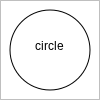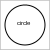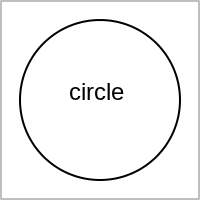## Clear¶

```void Clear(const OESystem::OEColor &color)
```

Appends a clear command to the display list of the OEImage object.

## DrawArc¶

```void DrawArc(const OE2DPoint &center, double bgnAngle, double endAngle,
```

Appends an arc drawing command to the display list of the OEImage object. See example in Figure: Example of drawing an arc.

## DrawCircle¶

```void DrawCircle(const OE2DPoint &center, double radius, const OEPen &pen)
```

Appends a circle drawing command to the display list of the OEImage object. See example in Figure: Example of drawing a circle.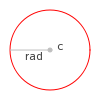Example of drawing a circle

## DrawCubicBezier¶

```void DrawCubicBezier(const OE2DPoint &bgn, const OE2DPoint &c1,
const OE2DPoint &c2, const OE2DPoint &end, const OEPen &pen)
```

Appends a cubic Bézier curve drawing command to the display list of the OEImage object. See example in Figure: Example of drawing a cubic Bezier curve.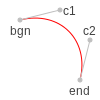Example of drawing a cubic Bezier curve

## DrawLine¶

```void DrawLine(const OE2DPoint &bgn, const OE2DPoint &end, const OEPen &pen)
```

Appends a line drawing command to the display list of the OEImage object. See example in Figure: Example of drawing a line.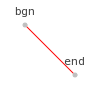Example of drawing a line

## DrawPath¶

```void DrawPath(const OE2DPath& path, const OEPen& pen);
```

Appends a path drawing command to the display list of the OEImage object. See example in Figure: Example of drawing a path.

## DrawPie¶

```void DrawPie(const OE2DPoint &center, double bgnAngle, double endAngle,
```

Appends a pie drawing command to the display list of the OEImage object. See example in Figure: Example of drawing a pie.

## DrawPoint¶

```void DrawPoint(const OE2DPoint &p, const OESystem::OEColor &color)
```

Appends a point drawing command to the display list of the OEImage object. See example in Figure: Example of drawing a point.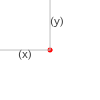Example of drawing a point

## DrawPolygon¶

```void DrawPolygon(const std::vector<OE2DPoint> &points, const OEPen &pen)
```

Appends a polygon drawing command to the display list of the OEImage object. See example in Figure: Example of drawing a polygon.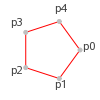Example of drawing a polygon

```void DrawQuadraticBezier(const OE2DPoint &bgn, const OE2DPoint &c,
const OE2DPoint &end, const OEPen &pen)
```

Appends a quadratic Bézier curve drawing command to the display list of the OEImage object. See example in Figure: Example of drawing a quadratic Bezier curve.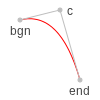Example of drawing a quadratic Bezier curve

## DrawRectangle¶

```void DrawRectangle(const OE2DPoint &tl, const OE2DPoint &br, const OEPen &pen)
```

Appends a polygon drawing command to the display list of the OEImage object. See example in Figure: Example of drawing a rectangle.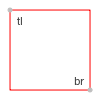Example of drawing a rectangle

## DrawText¶

```void DrawText(const OE2DPoint &c, const std::string &text, const OEFont &font,
double maxwidth=0.0)
```

Appends a text drawing command to the display list of the OEImage object. See example in Figure: Example of drawing a text.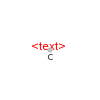Example of drawing a text

## DrawTriangle¶

```void DrawTriangle(const OE2DPoint &a, const OE2DPoint &b, const OE2DPoint &c,
const OEPen &pen)
```

Appends a triangle drawing command to the display list of the OEImage object. See example in Figure: Example of drawing a triangle.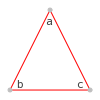Example of drawing a triangle

## GetHeight¶

```double GetHeight() const
```

Returns the height of the OEImage object.

## GetWidth¶

```double GetWidth() const
```

Returns the width of the OEImage object.

## IsEmpty¶

```bool IsEmpty() const
```

Returns whether or not the display list of the OEImage object is empty.

## Render¶

```bool Render(OEImageBase &image) const
```

Renders the image stored in the OEImage object by executing the stored drawing commands in the order in which they were issued.